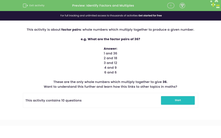# Identify Factors Pairs

In this worksheet, students will select all the factor pairs for a given number.Key stage:  KS 2

Curriculum topic:   Number: Multiplication and Division

Curriculum subtopic:   Identify Multiples and Factors

Popular topics:   Multiplication worksheets, Square Numbers worksheets, Factors worksheets

Difficulty level:#### Worksheet Overview

This activity is about factor pairs.

These are whole numbers that multiply together to produce a given number.Example

What are the factor pairs of 36?

1 and 36

2 and 18

3 and 12

4 and 9

6 and 6

These are the only whole numbers that multiply together to give 36.

Let's have a go at some questions now.### What is EdPlace?

We're your National Curriculum aligned online education content provider helping each child succeed in English, maths and science from year 1 to GCSE. With an EdPlace account you’ll be able to track and measure progress, helping each child achieve their best. We build confidence and attainment by personalising each child’s learning at a level that suits them.

Get started